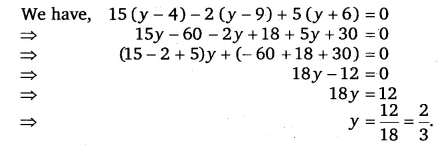# Simplify and solve the following linear equations: 15(y – 4) – 2 (y – 9) + 5(y + 6) = 0

Simplify and solve the following linear equations:
15(y – 4) – 2 (y – 9) + 5(y + 6) = 0